Next: 4.3 Carrier Energy Distribution Up: 4. Device Simulation and Previous: 4.1 Semiconductor Equations

Subsections

# 4.2 Parameter Modeling

The semiconductor equations discussed above show the basic relations between carrier distribution and the electrostatic potential. Two parameters, the mobility and the recombination rate were introduced, which require appropriate modeling. The physical phenomena which are crucial for modeling of these parameters will be discussed in the following.

## 4.2.1 Mobility

The derivation of the mobility originates from carrier relaxation times. The mobility is influenced by the lattice and its thermal vibrations, impurity atoms, surfaces and interfaces, interface charges and traps, the carriers themselves, the energy of the carriers, and other effects like lattice defects. Mobility models are used to make an estimation considering these effects and make simulations in continuous systems possible. Since exact derivations are too complex or just do not exist, empirical approaches are often used. Some of the commonly used approaches will be presented here.

A common method for modeling the mobility is the hierarchically encapsulation of the physical mechanisms. In this approach, the most fundamental mechanism is considered to be the lattice scattering dependence (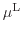) followed by the ionized impurity dependence (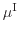). Especially in MOS devices, a surface correction () is of special importance. These three contributions classify the low-field mobility models. Modeling of high-field effects is introduced with a field dependence model (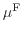). These contributions can be combined like in the MINIMOS mobility model , for example, which looks like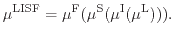(4.10)

The individual mechanisms are assumed to be independent of each other. All values resulting from mobility calculations are obviously different for electrons and holes. In contrast to the encapsulation approach (4.10) for the mobility calculation used in the MINIMOS model, the Lombardi model  combines three carrier mobility components using Matthiessen's rule. The components are derived from surface acoustic phonon scattering, from bulk carrier mobility, and from surface-roughness scattering. A similar expression has been used by Agostinelli  for holes, additionally accounting for interface charge and screened Coulomb scattering: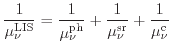(4.11)

Here, the phonon scattering component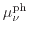combines scattering with bulk phonons, surface phonons, and fixed interface charges.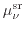includes the dependence of the surface-roughness scattering on the electric field orthogonal to the interface and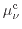models the screened Coulomb scattering. Other mobility models based on Matthiessen's rule have been developed, for example, by Darwish  or Neinhüs .

Effects like negative bias temperature instability  or hot carrier degradation (see Chapter 6) generate interface traps leading to interface charges. Modeling of their influence on the mobility is of special interest in reliability modeling . A mobility reduction due to oxide charges in inversion layers has been proposed by Sun et al.  as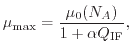(4.12)

where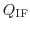is the surface oxide charge density (or treated as the interface charge density),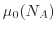is the impurity dependent mobility, and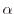is modeled as a parameter which depends weakly on the impurity. This mobility reduction model was extended by Wong et al.  to consider the influence of the trapped charges and of the interface states independently with separate coefficients. In the hot-carrier degradation simulations performed in this work, the model of Sun was applied in the following extended form as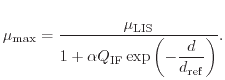(4.13)

The influence of the interface charge is here weighted with the negative exponent of the reciprocal interface distance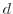to include the distance dependent influence of the charges with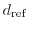as a model parameter.

The carrier mobility also depends on the carrier energy distribution. However, in the drift-diffusion model the electric field is usually employed. Simulation tools commonly differ between low- and high-field mobility and let the user select the models independently. The high-field mobility modeling approaches are often accomplished using the model presented by Caughey and Thomas . A slightly different version, suggested by Jaggi [148,149], is used in the MINIMOS mobility model,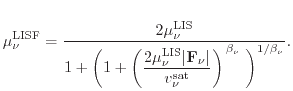(4.14)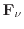describes the driving force, which is the gradient of the quasi-Fermi level and proved to give better results then just the electric field,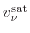is the saturation velocity, and the value of the coefficient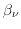is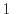for holes and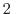for electrons. The impact of this field dependence is visible in the mobility distribution of the sample device shown in Fig. 4.3.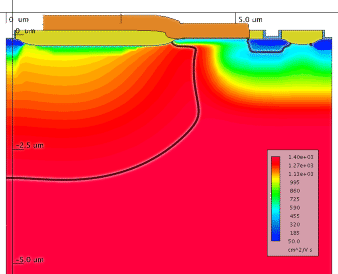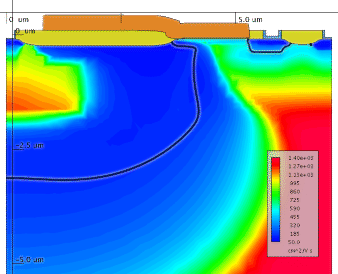To illustrate the impact of mobility models a comparison of simulation results with constant mobility, a low-field mobility model, and a high-field mobility model are shown in Fig. 4.4. Comparing the constant mobility and the low-field mobility model, one can see that the shape changes only slightly, but the total current is reduced significantly for the low-field mobility model (note the multiplication factors in the legend). This is caused by the reduction of the mobility, especially near the surface, which can be clearly seen in Fig. 4.3. The transconductance is only slightly influenced, whereas for the high-field mobility model, a strong reduction can be observed. Hence, for the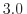V curve, it can be seen clearly that the current initially increases steeply with the drain voltage, but immediately flattens, since the mobility reduction becomes effective. The effective mobility distribution at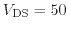V can be seen in Fig. 4.3.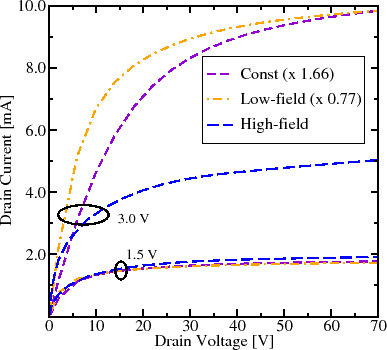Carrier mobility modeling has been investigated since the beginning of semiconductor engineering, and there are still new models published . However, all approaches in the drift-diffusion model which incorporate the influence of carriers that are not in thermal equilibrium basically rely on the electric field. Changes in the electric field therefore directly change the calculated mobility (see Fig. 4.5), whereas the distribution function and therefore the carrier temperature do not change immediately. Mobility models in higher-order transport models can use more information from the distribution function. In energy-transport, for example, the carrier temperature can be used as a parameter. As a consequence effects like the velocity overshoot can be described.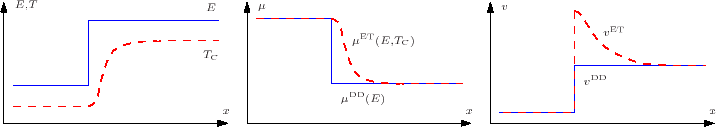## 4.2.2 Carrier Generation and Recombination

The recombination rate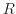was formally introduced in the drift-diffusion equations (4.5) and (4.6) by splitting the continuity equation into two individual parts for electrons and holes. From a physical point of view this term includes the generation and the recombination of electron-hole pairs. In thermal equilibrium carrier generation and recombination are balanced and the carrier concentrations are given by their equilibrium values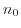and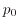(). The net recombination rate therefore vanishes. An excess number of carriers leads to an increased recombination, a low carrier concentration leads to an increased generation. The generation and recombination processes contributing to the total effective net generation rate are based on different physical effects which are modeled independently of each other. The separately evaluated models add up to the total net recombination rate. The resulting rate is used to complete the continuity equations (4.5) and (4.6).

One important generation/recombination process is the well-known Shockley-Read-Hall (SRH) mechanism [152,153] which describes a two-step phonon transition. One trap level which is energetically located within the band-gap is utilized. Four partial processes can be separated: the capture and the emission of both, electrons and holes, on the trap level. Balance equations can be formulated for the trap occupancy function. In the stationary case the rates for electrons and holes are equal. The trap occupancy function can then be eliminated and the SRH generation rate results in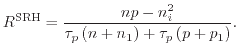(4.15)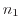and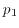are auxiliary concentrations depending on the energy level of the traps andand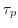are carrier lifetimes for electrons and holes, respectively.

In MOS devices SRH generation especially influences the bulk current. In n-channel devices, for example, holes generated at the pn-junction are attracted by the low bulk potential leading to the bulk current. The influence can be easily observed in device simulation since models can be switched on or off allowing to deactivate SRH. Fig. 4.6(a) shows the hole current flow and the SRH generation rate in the sample device and in Fig. 4.6(b) the current components on the bulk contact are compared with and without SRH enabled.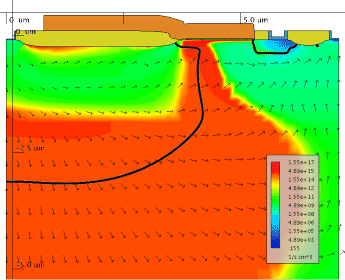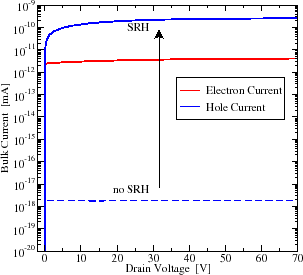The SRH model is not restricted to the description of capture and emission of carriers in the bulk, it can also be extended to determine the occupancy of interface traps . Like most interface related mechanisms this is especially relevant for MOS devices. Simulations of charge pumping (CP) measurements , which are used to determine interface trap distributions, require appropriate modeling of trapping and de-trapping effects of carriers in interface traps. In a CP-simulation the measurement procedure is replicated, by performing a transient simulation for each gate pulse level (Fig. 4.7(a)).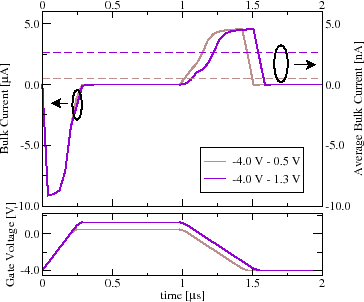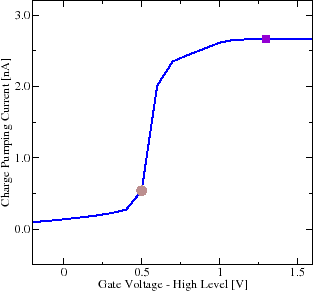In contrast to the stationary SRH formulation shown in (4.15), time dependent simulations require to capture the transient behavior of the occupancy function . The final charge pumping curve can be constructed by extracting the mean bulk current of the simulations for every single gate pulse (Fig. 4.7(b)).

In addition to the two-particle SRH mechanism there are other important generation mechanisms to mention: the Auger and the impact-ionization process, both of which are three-particle processes. The impact-ionization process, a very important mechanism when considering hot-carrier processes, is discussed in detail in Chapter 5. The Auger generation is a pure generation process. The energy required for carrier generation is delivered by a third higher-energetic electron or hole. In the Auger process, additionally the excess energy which is available after a recombination process is transferred to a third particle electron or hole. Modeling of this process can be achieved by defining rates for each partial process. In the stationary case the rate evaluates to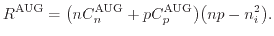(4.16)

The coefficients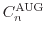and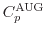have a weak dependence on the temperature [157,158]. However, the coefficients are often assumed to be constant.

There are various other generation and recombination mechanisms which have not been mentioned here. Among them are, just to mention a few, the direct recombination which is crucial for direct bandgap semiconductors, the direct  and trap assisted  band-to-band tunneling in high field regions, and optical generation .

## 4.2.3 Thermal Modeling

Many physical properties of semiconductor devices strongly depend on the lattice temperature. Especially in high-voltage and power devices, the self-heating of the device is of special importance and the temperature distribution within a device is needed to estimate the device behavior at operating conditions. Regions of special interest are associated to high-current densities. In MOSFETs, these regions are commonly at the drain end of the channel, in the drain extensions and drift zones, and at corners .

For the definition of a reference temperature and for the dissipation of the generated heat, the simulation domain must be connected to one or more external reference temperatures or heat sinks. This connection is modeled in terms of thermal contacts, which have assigned fixed temperatures and are connected to the simulation domain via thermal resistances. It is also important to consider that the heat flow in a semiconductor device extends to areas that are electrically less important. Hence, the simulation domain usually has to be extended in comparison to iso-thermal simulations . At the simulation domain boundaries representing symmetries in the device, Dirichlet conditions are used. For a proper modeling of corner effects, a three-dimensional simulation has to be used [163,164].

The lattice temperature distribution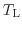is modeled using the heat conduction equation(4.17)

where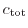is the total heat capacity and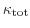the total thermal conductivity. Both parameters include contributions from the lattice, the electron, and the hole subsystem. The temperature differences in the lattice lead to an additional driving force on the carriers which is already considered in the last terms in the current equations (4.7) and (4.8).

Different approaches of modeling the heat generation rate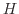have been proposed. The most simple approach considers only the Joule heat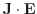. A more accurate model according to Adler  describes the generated heat using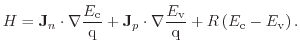(4.18)

Here, the energy dissipation due to recombination is considered. A more rigorous treatment to thermal generation is given by Wachutka .

Transient simulations including thermal modeling were performed using the sample device. The lower bulk contact is linked with a thermal resistance to the ambient temperature of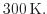In this simulation, the drain voltage is raised linearly from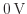to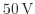using two different slopes. The temperature distributions at the end of the two voltage ramps are depicted in Fig. 4.8. At the end of the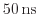slope a rapid increase of the temperature near the birds beak can be observed.

Figure 4.8: Temperature distribution in the sample device with the gate biased toV at the end of a linearly increase of the drain voltage from 0 to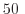V inns (a) and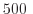ns (b).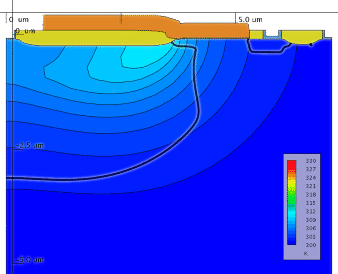(a)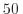ns slope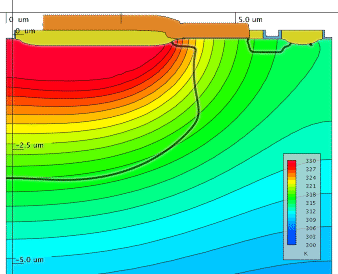(b)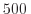ns slope